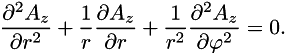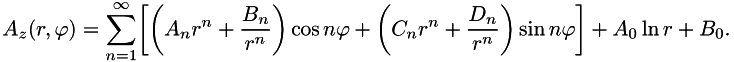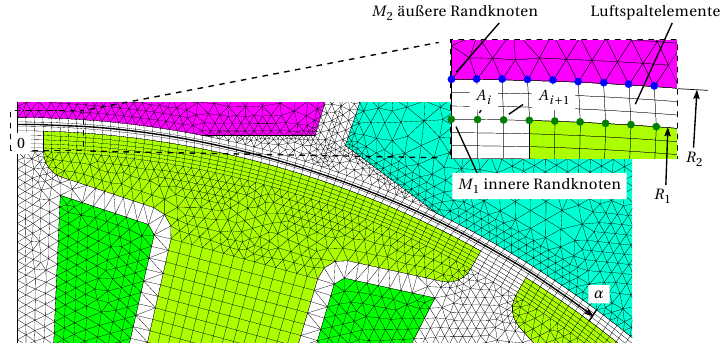# Air-gap field and force density¶

The numerical field calculation based on the finite element method encounters recognition and discretization errors. Combined methods are known to describe geometrically simple sub-range of the machine, e.g. the air gap relevant for force calculation, analytically based on the numerically calculated vector potentials at the edges . The force calculated based on the Maxwell stress will still depend on discretization, but not on local influences, such as the chosen calculation path through the elements and the shape of these elements . The analytical evaluation also allows to directly specify the amplitudes of the air gap induction waves and the radial force waves.

According to , in two-dimensional problems in the air-gap of an electric machine, the magnetic vector potential A = (0,0,Az) is described by the Laplace equation in polar coordinates (r,φ): thusThe general harmonic solution exists:The Fourier coefficients A, B, D, C can be determined when the vector potentials Ai are known at nodes M1 and M2 on the boundaries of a superelement having an inner radius R1 and an outer radius R2 ; see Abb. 1 and references [1,2,3].The FEMAG script language provides the following routines for calculating the air-gap field and derived values according to the method described above.

The following prerequisites are met:

• Arrangement in the polar reference system (r-phi-system)

• Area with constant permeability

• Superelement defined by a closed node chain (see Objects in FEMAG )

• Ring or ring segment (usually the air-gap) bounded by outer and inner circular node-chains that have constant radii and the same centre.

## Literatur¶

 Chao, B. and Chen, S. X. and Liu, Z. J. and Low, T. S.: Electromagnetic field analysis in rotational electric machines using finite element-analytical hybrid method. In: IEEE Transactions on Maggnetics 30 (Nov. 1994) No. 6, pp. 4314–4316

 Chao, B. and Liu, Z. J. and Low, T. S.: Analysis of unbalanced-magnetic-pulls in hard disk drive spindle motors using a hybrid method. IEEE Transactions on Magnetics 32 (1996), No. 5, pp. 4308-4310

 Low, T. S. and Bi, C. and Liu, Z. J.: A hybrid technique for electromagnetic torque and force analysis of electric machines. COMPEL: The International Journal for Computation and Mathematics in Electrical and Electronic Engineering 16 (January 1997), No. 3, pp. 191-205

 Krotsch, J.: Numerisch-analytische Berechnung des Luftspaltfeldes - Realisierung und Anwendung an Beispielen, FEMAG-Anwendertreffen 2015, Leutkirch

## Command description¶

Function: N, stat = evalBNP_fourcoeff(x, y)

Calculation of the Fourier coefficients of the harmonic solution of Laplace’s equation to describe the field variation in the superelement addressed by the point (x,y), starting from the vector potentials of the boundary nodes of the superelement. Usually, the superelement corresponds to the air gap of an electric machine. (BNP = Boundary Node potential).

Parameter

x,y Coordinates of test point in global units

Return value
N Number of Fourier coefficients (= largest ordinal)
stat Status of the execution (0 = error; 1 = no error)

Note

The return variables do not need to be specified in full when the call is made, but always in the order defined above.

Example:

```N = evalBNP_fourcoeff(x,y)
```

or

```N,stat = evalBNP_fourcoeff(x,y)
```

Function: Az, gamma, stat = evalBNP_Avecpot(r, n)

Returns the amplitude Az and the Fourier angle gamma of the nᵗʰ harmonic vector-potential component along the perimeter with radius r.

Parameter
r Radius in global units; R1 < r < R2
n Harmonic order
Return values
Az Amplitude of the vector-potential component of harmonic order n at radius r
gamma Phase-angle of the vector-potential component
stat Status of the execution (0 = error; 1 = no error)

Example:

```Az = evalBNP_Avecpot(r,n)
printf("Amplitude der %d-ten Ordnung: %g",n,Az)
```

Function: az, stat = evalBNP_vecpot(r, phi)

Returns the vector potential az at the point r, phi within the superelement (air-gap).

Parameter
r Radius in global units; R1 < r < R2
phi Azimuthal angle of test point [rad]
Return value
az Vector potential at the point (r,phi)
stat Status of the execution (0 = error; 1 = no error)

Returns the amplitudes ABrad, ABphi and the Fourier angles gBrad or gBphi of the nᵗʰ space-harmonic component of the flux-density distribution along the circumference with radius r.

Parameter
r Radius in global units, with R1 < r < R2
n Azimuthal angle of test point [rad]
Return value
ABphi Amplitude of the nᵗʰ space-harmonic component of tangential flux-density [T]
gBphi Phase angle of the nᵗʰ space-harmonic component of tangential flux-density [rad]
stat Status of the execution (0 = error; 1 = no error)

Function: Brad, Bphi, stat = evalBNP_induc(r, phi)

Returns the radial component Brad and the tangential component Bphi of the flux-density at point r, phi.

Parameter
r Radius in global units, with R1 < r < R2
phi Azimuthal angle of test point [rad]
Return value
Bphi Tangential component of flux-density [T] at the point (r,phi)
stat Status of the execution (0 = error; 1 = no error)

Returns the amplitudes Afrad, Afphi and the Fourier phase-angles gfrad or gfphi of the force-density wave (‘Maxwell voltage’) of the space-harmonic order number n along the perimeter with radius r.

Parameter
r Radius in global units; R1 < r < R2
n Harmonic order
Return values
Afrad Amplitude of the nᵗʰ space-harmonic component of the radial component of the force-density wave along the perimeter with radius r [N/m²]
Afphi Amplitude of the nᵗʰ space-harmonic component of the tangential component of the force-density wave along the perimeter with radius r [N/m²]
gfphi Phase-angle of the nᵗʰ space-harmonic component of the tangential component of the force-density wave [rad]
stat Status of the execution (0 = error; 1 = no error)

Function: frad, fphi, stat = evalBNP_forcedens(r, phi)

Returns the radial component frad and the circumferential component fphi of the force density (Maxwell stress) at point (r,phi).

Parameter
r Radius in global units; R1 < r < R2
phi Azimuthal angle of test point [rad]
Return value
fphi Tangential component of force density at the point (r,phi) [N/m²]
stat Status of the execution (0 = error; 1 = no error)

Function: T, Fx, Fy, stat = evalBNP_forcetorq( )

Returns the torque T and the resulting forces Fx and Fy exerted on the area enclosed by the superelement (usually the air-gap). The forces are always calculated, regardless of the model domain.

Return value
T Total torque for the whole machine length [Nm]
Fx x-component of the total force for the whole machine length [N]
Fy y-component of the total force for the whole machine length [N]
stat Status of the execution (0 = error; 1 = no error)

## Example:¶

This script fragment performs a linear finite element analysis, calculates the air-gap field sizes based on the edge potentials of the air-gap, outputs them as distribution and amplitude spectra in files. The forces and torque are calculated vs. the rotor rotation position and also output in a file.

```file1=io.open("BNP_ForceTorque.txt","w+")

for i=1,90 do

calc_field_single(1,actual,0.01)     -- lineare FEA

r = 34.2
x,y = pd2c(r,0.5)
Nk = evalBNP_fourcoeff(x,y)         -- Harmonische Lösung der PDGL im Luftspalt

if (i==1) then                           -- Ausgabe Verteilungen für die Ausgangslage
file2=io.open("BNP_Distr.txt","w+")
for j=0,360 do
phi = j/180*math.pi
Az = evalBNP_vecpot(r,phi)
Br,Bt = evalBNP_induc(r,phi)
fr,ft = evalBNP_forcedens(r,phi)
file2:write(string.format("
end
io.close(file2)

file2=io.open("BNP_Four.txt","w+")  -- Ausgabe des Amplitudenspektrumf für die Ausgangslage
for j=0,Nk do
AAz = evalBNP_Avecpot(r,j)
ABr,ABt = evalBNP_Ainduc(r,j)
Afr,Aft = evalBNP_Aforcedens(r,j)
file2:write(string.format("
end
io.close(file2)
end

phi=dphi*i                    -- Ausgabe des Drehmoments und der Kräfte über der Drehstellung
M,Fx,Fy = evalBNP_forcetorq()
file:write(string.format("

rotate(r,1.0,inside,increment)     -- Drehung des Rotos

end

io.close(file1)
```

For a full running sample script, see the script example Luftspaltfeld numerisch-analytisch.